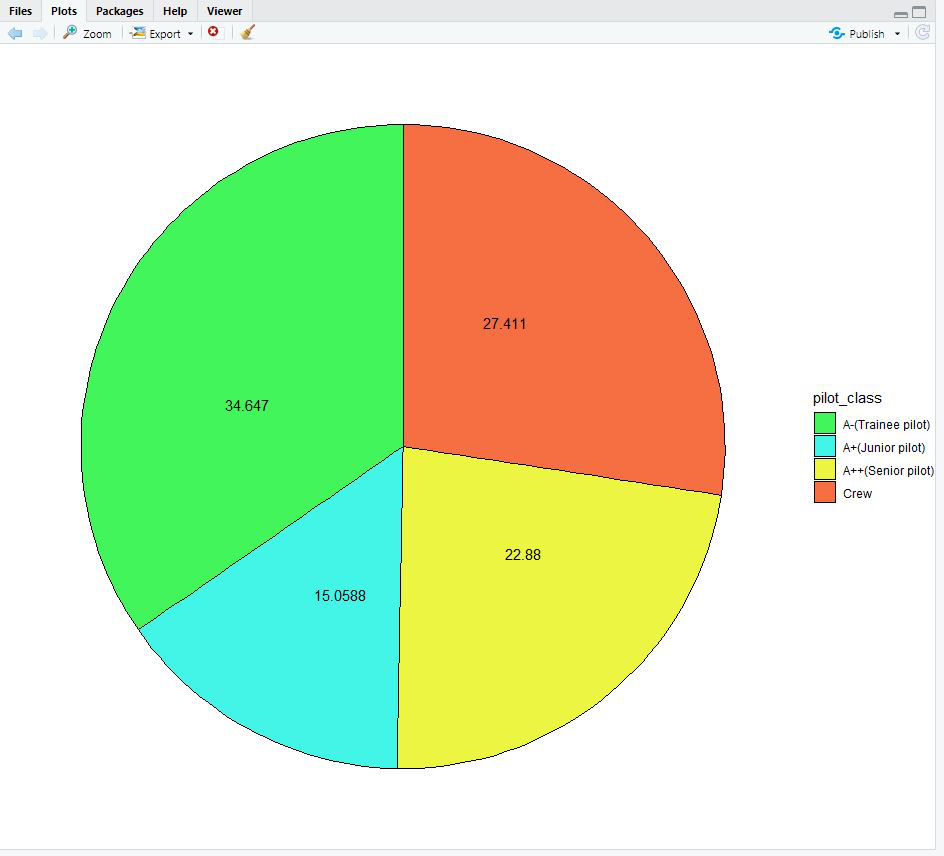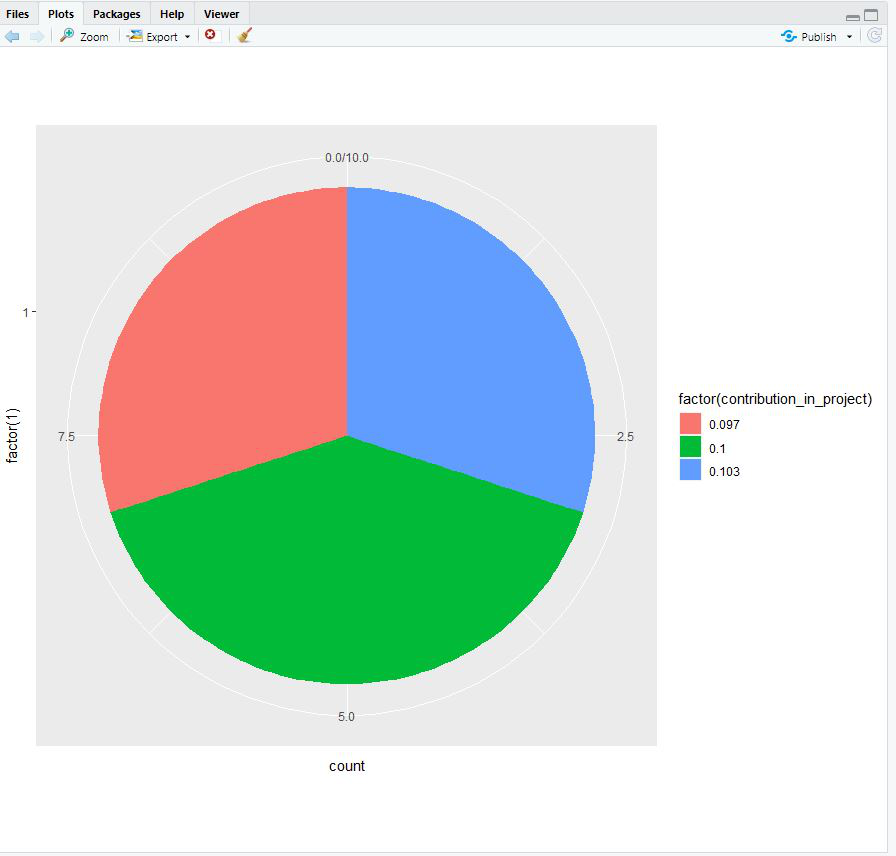# Pie Charts in R using ggplot2

• Last Updated : 25 Feb, 2021

A Pie Chart or Circle Chart is a circular statistical graphical technique that divides the circle in numeric proportion to represent data as a part of the whole. In Circle Chart the arc length of each slice is proportional to the quantity it represents. Pie charts are very widely used in the business world and the mass media to understand trends.

Approach

• Import module
• Create data
• Arrange the rows of a data frame by the values of selected columns.
• Mutate to create a new variable from a data set
• Plot pie chart
• Display plot

Example 1:

## R

 `library``(ggplot2)``library``(dplyr)`` ` `count.data <- ``data.frame``(``    ``pilot_class = ``c``(``"A++(Senior pilot)"``, ``"A+(Junior pilot)"``, ``"A-(Trainee pilot)"``, ``"Crew"``),``    ``n = ``c``(389, 256, 589, 466),``    ``proportion = ``c``(22.88, 15.0588, 34.647, 27.411)``)`` ` `count.data`` ` `count.data <- count.data %>%``    ``arrange``(``desc``(pilot_class)) %>%``    ``mutate``(lab.ypos = ``cumsum``(proportion) - 0.6*proportion)`` ` `count.data`` ` `mycols <- ``c``(``"#42f55a"``, ``"#42f5e6"``, ``"#ecf542"``, ``"#f56f42"``)`` ` `ggplot``(count.data, ``aes``(x = ``""``, y = proportion, fill = pilot_class)) +``    ``geom_bar``(width = 1, stat = ``"identity"``, color = ``"black"``) +``    ``coord_polar``(``"y"``, start = 0)+``    ``geom_text``(``aes``(y = lab.ypos, label = proportion), color = ``"black"``)+``    ``scale_fill_manual``(values = mycols) +``    ``theme_void``()`

Output:Example 2:

## R

 `Name_of_student <- ``c``(``"Ankit"``, ``"Jack"``, ``"Prakhar"``, ``"Madahav"``, ``"shef"``, ``            ``"sama"``, ``"karthik"``, ``"Ritwik"``, ``"Agnim"``, ``"Harsh"``)`` ` `contribution_in_project <- ``c``(.103, .097, .103, .103,.097, .097, .10,.10,.10,.10 )`` ` `top_ten_contributors <- ``data.frame``(Name_of_student,contribution_in_project)``top_ten_contributors`` ` `pie_2 <- ``ggplot``(top_ten_contributors, ``aes``(x = ``factor``(1), fill = ``factor``(contribution_in_project))) +``    ``geom_bar``(width = 1)`` ` `pie_2 + ``coord_polar``(theta = ``"y"``)`

Output:My Personal Notes arrow_drop_up